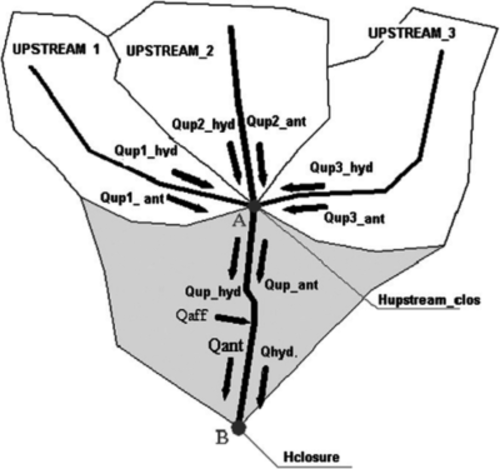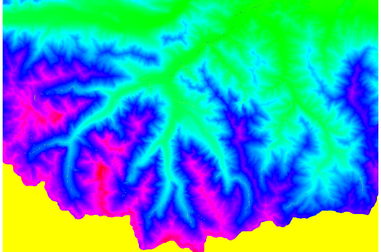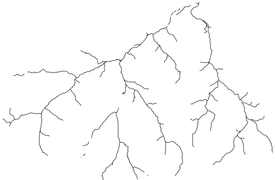## NAME

r.green.hydro.theoretical - Calculate the hydropower energy potential for each basin starting from discharge and elevation data. If existing plants are available it computes the potential installed power in the available part of the rivers.

## KEYWORDS

raster, hydropower, renewable energy

## SYNOPSIS

r.green.hydro.theoretical
r.green.hydro.theoretical --help
r.green.hydro.theoretical [-d] elevation=name discharge=name [rivers=name] [lakes=name] threshold=string [basins=name] [stream=name] output=name [--overwrite] [--help] [--verbose] [--quiet] [--ui]

### Flags:

-d
Debug with intermediate maps
--overwrite
Allow output files to overwrite existing files
--help
Print usage summary
--verbose
Verbose module output
--quiet
Quiet module output
--ui
Force launching GUI dialog

### Parameters:

elevation=name [required]
Name of input elevation raster map
discharge=name [required]
Name of input river discharge raster map [m3/s]
rivers=name
Name of input vector map
Name of river network input vector map
lakes=name
Name of input vector map
Name of lakes input vector map
threshold=string [required]
Minimum size of exterior watershed basin
Default: 0
basins=name
Name of basin map obtained by r.watershed
stream=name
Name of stream map obtained by r.watershed
output=name [required]
Name of output vector map with basin potential [MWh]

## DESCRIPTION

r.green.hydro.theoretical calculates for each basin the theoretical maximum hydropower energy potential based on the input raster files with discharge data along a river network and digital terrain elevation models of the considered region.
If there are already existing plants, the function computes the potential installed power in the available parts of the rivers.
This module returns two output vector maps with the available river segments and the optimal position of the plants with their potential maximum powers, intakes and restitutions.
In this module the output is the theoretical maximum hydropower energy that can be converted in the ideal case without considering the efficiency of energy transformation.

## NOTES

The required inputs are the elevation raster map, the river discharge raster map and the value for the minimum size of the exterior watershed basin.
You can optionally add vector maps of existing river networks and lakes that will be considered in the calculation and make the output more realistic.
Instead of the minimum size of the exterior watershed basin you can also enter the basin and stream maps created by r.watershed.

## EXPLANATION

The maximum potential hydropower establishes the theoretical maximum of energy that the study basin can produce assuming that all water resources are used to produce energy.
In real life this situation does not arise, because of environmental flows, other water uses and economic cost analysis.

The underlying methods of calculation explained below are based on the considerations and formulas used in the article "A GIS-based assessment of maximum potential hydropower production in La Plata Basin under global changes" written by M. Peviani, I. Popescu, L. Brandimarte, J.Alterach and P. Cuya.

The maximum potential hydropower at subbasin scale can be computed as the sum of two components:

- upstream subbasin potential           - subbasin own potential

According to the general schematization in the figure below, point A is the closure point of the upstream subbasins (named UPSTREAM 1, UPSTREAM 2 and UPSTREAM 3).
The three rivers belonging to the three upstream basins merge into the common river of the downstream basin in point A (named Upstreamclosure point).
The downstream basin is bounded by the two closure points A and B.
The scheme divides the subbasins in upper portions, whose energy production is only given by their own potential and a lower portion, whose energy production is the sum of the two components, own potential and the potential given by the flow coming from the upper portions.Subbasin scheme to calculate maximum potential hydropower

The maximum potential hydropower for the upstream subbasins is given by the energy formula applied to the upstream inflows:

Eown_max = conv * g * η * Qup_hydro * (Hmean - Hclosure)
where conv is the adimensional conversion factor to calculate energy in GWh (conv = 0.00876);
g is a gravity constant (9.81 m/s2);
η is the overall electrical efficiency;
Qup_hydro is the mean annual discharge at the closure section for the upstream subbasin;
Hmean is the mean elevation of the upstream subbasin calculated from the hypsographic curve, using the statistical tool of Arc-GIS;
Hclosure is the elevation at the closure point (point A in the figure);

The downstream lower subbasin (between point A and B in the figure) has both energy components: the potential from the upstream subbasins and its own potential. The own potential is calculated taking into account the discharge coming from the sides of the downstream lower subbasin and the difference between the elevation of the lower subbasin and the elevation at the closure point (point B in the figure):

Eown_max = conv * g * η * Qaff * (Hmean - Hclosure)
where Qaff is the afferent discharge (own lower subbasin discharge). The afferent discharge is the difference of the discharge observed at the closure section (point B in the figure) and the sum of the upstream discharges;
Hmean is the elevation of lower subbasin;
Hclosure is the elevation at closure point (point B in the figure);

The upstream component to the potential of the downstream lower subbasin is calculated taking into account the discharge coming from the upstream subbasins and the difference between the elevation of the upstream closure point (point A in the figure) and the elevation at the basin closure (point B in the figure):

Eup_max = conv * g * η * ∑Qup_hydro * (Hup_closure - Hclosure)
where Qup_hydro is the sum of the mean annual discharges coming from the upstream subbasins;
Hup_closure is the elevation at the upstream closure point (point A in the figure);
Hclosure is the elevation at closure point (point B in the figure);

The total maximum hydropower potential of the overall given basin is the sum of the different contributions computed at the subbasin level:

Etotal_max = Eown_max + Eup_max

## EXAMPLES

EXAMPLE 1

This example is based on the case-study of the Gesso and Vermenagna valleys located in the Piedmont Region, in South-West Italy, close to the Italian and French border.

In the map below you can see the input files elevation and natural discharge.input raster map with elevation and natural discharge

For a faster run of this example, the input maps elevation and discharge are limited to the section that can be modified by r.green.hydro.theoretical using the code

To create the map of this example, you can type in the following code in the command console or if you prefer you can only type in the main function r.green.hydro.theoretical in the console and specify the other parameters of the code like elevation or discharge by using the graphical user interface.
`r.green.hydro.theoretical elevation=elevation discharge=naturaldischarge rivers=streams lakes=lakes basins=basin stream=stream output=out`

In the map below, you can see the output vector map with the basin potential.output vector map with basin potential

r.green.hydro.discharge
r.green.hydro.delplants
r.green.hydro.optimal
r.green.hydro.recommended
r.green.hydro.structure
r.green.hydro.technical
r.green.hydro.financial

## AUTHORS

Giulia Garegnani (Eurac Research, Bolzano, Italy), Manual written by Sabrina Scheunert.

## SOURCE CODE

Available at: r.green.hydro.theoretical source code (history)

Latest change: Monday Jan 30 19:52:26 2023 in commit: cac8d9d848299297977d1315b7e90cc3f7698730

© 2003-2023 GRASS Development Team, GRASS GIS 8.3.1dev Reference Manual